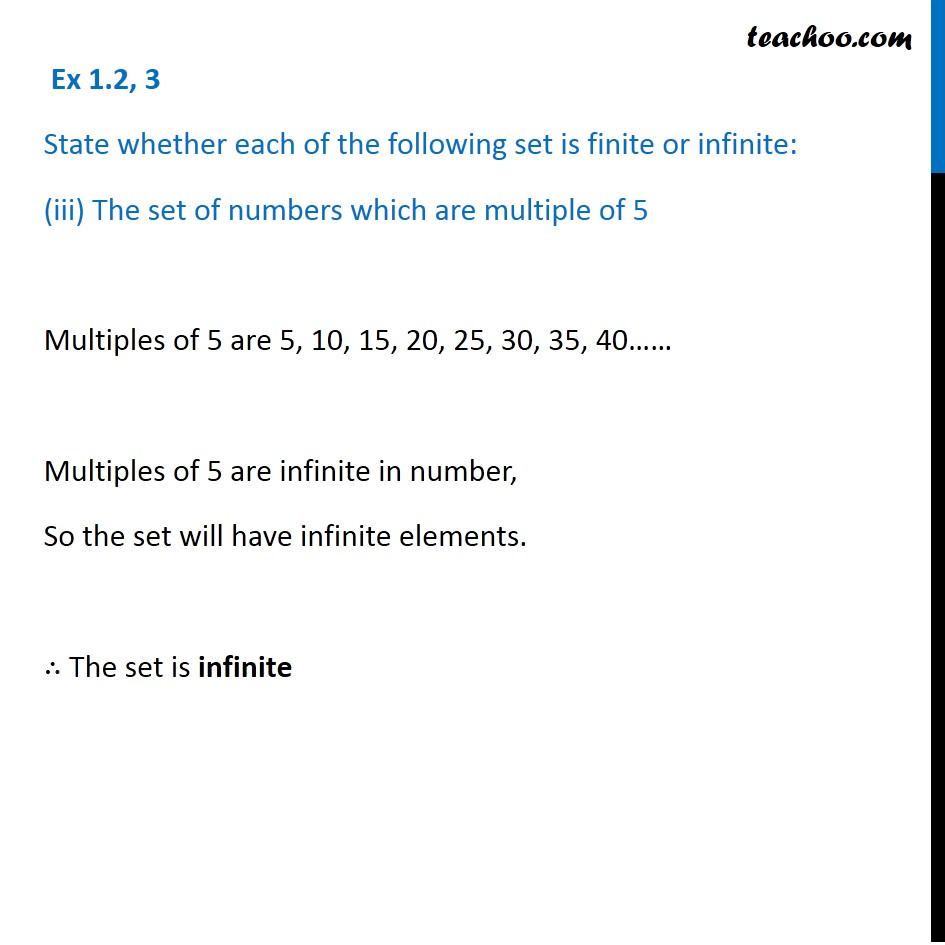1. Chapter 1 Class 11 Sets (Term 1)
2. Serial order wise
3. Ex 1.2

Transcript

Ex 1.2, 3 State whether each of the following set is finite or infinite: (iii) The set of numbers which are multiple of 5 Multiples of 5 are 5, 10, 15, 20, 25, 30, 35, 40…… Multiples of 5 are infinite in number, So the set will have infinite elements. ∴ The set is infinite